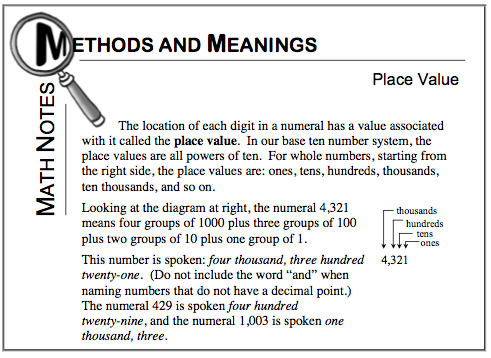### Home > MC1 > Chapter 10 > Lesson 10.1.1 > Problem10-14

10-14.

Convert each fraction to an equivalent decimal accurate to the specified degree.

1. $\frac { 3 } { 8 }$ (to the nearest hundredth)

2. $\frac { 1 } { 6 }$ (to the nearest tenth)

3. $\frac { 4 } { 3 }$ (to the nearest thousandth)

4. $\frac { 4 } { 13 }$ (to the nearest hundredth)

For parts (a) through (d), refer to the Math Notes box excerpt below. Can you change the fractions to decimals?

$\frac { 3 } { 8 } = 3 \div 8 = 0.375$

If you need to review your knowledge of place value, refer to the Math Notes box below as well.The answer to part (a) is 0.38 and the answer to part (b) is 0.2. Can you find the answers to parts (c) and (d)?